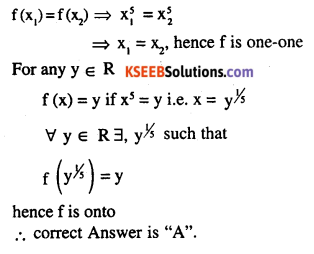# 2nd PUC Maths Question Bank Chapter 1 Relations and Functions Ex 1.2

Students can Download Maths Chapter 1 Relations and Functions Ex 1.2 Questions and Answers, Notes Pdf, 2nd PUC Maths Question Bank with Answers helps you to revise the complete Karnataka State Board Syllabus and score more marks in your examinations.

## Karnataka 2nd PUC Maths Question Bank Chapter 1 Relations and Functions Ex 1.2

### 2nd PUC Maths Relations and Functions NCERT Text Book Questions and Answers Ex 1.2

Question 1.
Show that the function f : R0 → R0 defined by $$f(x)=\frac{1}{x}$$  is one-one and onto, where R0 is the set of all non-zero real numbers. Is the result true, if the domain R0 is replaced by N with co-domain being same as R0?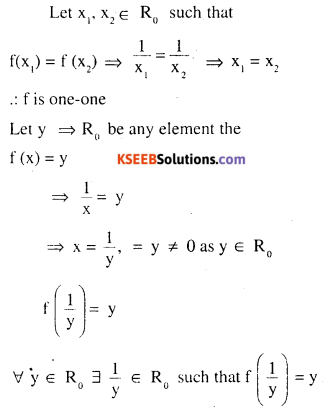f : N → R0,  then the function will remains one – one however mot onto.
Here the range of $$\mathrm{f}=\left\{1, \frac{1}{2}, \frac{1}{3}, \ldots \ldots .\right\} \neq \mathrm{R}_{\mathrm{e}}$$Question 2.
Check the injectivity and surjectivity of the following functions:
(i) f : N → N given by f (x) = x2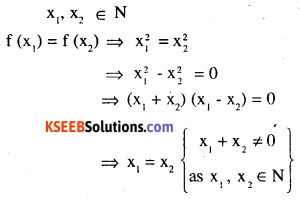hence f is injective, for some elements like, 2, 3 etc has no preimage in N such that
f (x) = 2 hence not surjective.

(ii) f : Z → Z given by f(x) = x2
f is not one-one or injective as f (1) = f (-1) ⇒ 1 = -1
f is not on to or surjective as $$f(x)=2 \Rightarrow x=\sqrt{2} \notin Z$$

(iii) f : R → R given by f(x) = x2
f is not injective
as f (x1) = f (x2) ⇒ x1= ± x2
Range off does not contain any negative real. Hence not surjective.

(iv) f : N → N given by f(x) = x2
Let x1, x2 ∈ N
f(x1) = f(x2) ⇒ $$x_{1}^{3}=x_{2}^{3}$$
hence one-one
Range of f= {1,8,27,…… } ≠ N
f is not surjective.(v) f : Z → Z given by f (x) = x3
Let x1, x2 ∈ Z
\begin{aligned} f\left(x_{1}\right)=f\left(x_{2}\right) & \Rightarrow x_{1}^{3}=x_{2}^{3} \\& \Rightarrow x_{1}=x_{2} \end{aligned}
f is one-one or injective
Range of f = {0, ±1, ±8, ±27,…………….. } ≠Z
f is not surjective

(vi) f : R → R given by f (x) = x3Question 3.
Prove that the Greatest Integer Function f : R → R, given by f(x) = [x], is neither one-one nor onto, where [x] denotes the greatest integer less than or equal to x.
∀ x ∈ [0, 1)
f (x) = [x] = 0
f is not one-one, or injective
ex: $$0, \frac{1}{2} \in R \Rightarrow f(0)==0$$
$$f\left(\frac{1}{2}\right)=\left[\frac{1}{2}\right]=0$$
Range of f is the set of integers ≠ R hence f is not onto.

Question 4.
Show that the Modulus Function f : R → R, given by f (x) = | x |, is neither one-one nor onto, where | x | is x, if x is positive or 0 and | x | is – x, if x is negative.
Let x1, x2 ∈ R f (x.) = f (x1)
\begin{aligned}f\left(x_{1}\right)=f\left(x_{2}\right)&\Rightarrow\left|x_{1}\right|=\left|x_{2}\right| \\& \Rightarrow x_{1}=\pm x_{2} \end{aligned}
$$|-2|=|+2|=2$$hence f is not one-one.
Range of functions is only non negative real numbers
Range of f = {0, ∞) ≠ R
∴ f is not onto.Question 5.
Show that the Signum Function f : R → R, given by
$$f(x)=\left\{\begin{array}{ll}{1} & {\text { if } x>0} \\{0} & {\text { if } x=0} \\{-1} & {\text { if } x<0}\end{array}\right.$$
is neither one-one nor onto.
f (x) = 1 ∀ x ∈ [0, ∞ )
hence f is not one-one Range of f = {-1,0, 1} hence f is not onto.

Question 6.
Let A = {1, 2, 3}, B = {4, 5, 6, 7} and let f = {(1,4), (2,5), (3,6)} be a function from A to B. Show that f is one-one.
x1, x2 ∈ A, f (x1) = f (x2)
⇒ x1 = x2
∴ hence one-one
f (1) = 4, f (2) = 5, f (3) ≠ R, hence f is one-one.

Question 7.
In each of the following cases, state whether the function is one-one, onto or bijective. Justify your answer.
(i) f : R → R defined by f(x) = 3 – 4x
Let x1 , x2 ∈ R
f (x1) = f (x2) ⇒ 3- 4x1, = 3- 4x2
⇒ x1 = x2 hence one-one function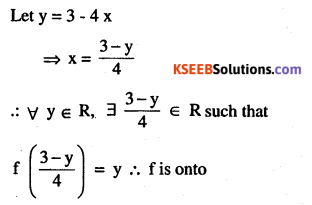hence f is not onto

(ii) f : R→ R defined by f(x) = 1 + x2
Let x1, x2 ∈ R
f (x1) = f (x2)
⇒ 1 + x12 = 1 + x22
⇒ x1 = ±x2
∴  f is not one-one
Also range of f contains only real numbers which is greater than or equal to 1≠ R
∴ f is not onto hence f is not bijective.Question 8.
Let A and B be sets. Show that
f : A x B → B x A such that
f (a, b) = (b, a) is bijective function.
Let (p, q), (r, s) g A x B f (p,q) = f (r, s)
⇒ (q, p) = (s, r) ⇒ q = s and p = r
⇒ (p, q) = (r, s), hence one-one
∀ (b, a) ∈ B x A, 3 (a, b) ∈ A x B
such that f (a, b) = f (b, a), hence on to hence f is bijective function

Question 9.
Let f : N → N be defined by
$$\mathbf{f}(\mathbf{n})=\left\{\begin{array}{c} {\frac{\mathbf{n}+\mathbf{1}}{2}, \text { if } \mathbf{n} \text { is odd }} \\{\mathbf{All} \mathbf{n} \in \mathbf{N}} \\{\frac{\mathbf{n}}{2}, \text { if } \mathbf{n} \text { is even }}\end{array}\right.$$
$$f(2 n-1)=\frac{2 n-1+1}{2}=n$$
$$f(2 n)=\frac{2 n}{2}=n$$
f (2n – 1) = f (2n) = n, but 2n -1 ≠ 2n
en, f (3) = 2, f (4) = 2, but 3 ≠ 4
hence f is not one-one
Here range of f = N
as for any N ∈ N, ∋ 2n ∈ N such that
f(2n) = n
hence f is on to. But it is not bijectiveQuestion 10.
Let A = R – {3} and B = R – {1}. Consider the function f : A → B defined by $$f(x)=\left(\frac{x-2}{x-3}\right)$$. is f one-one onto? justify your answer.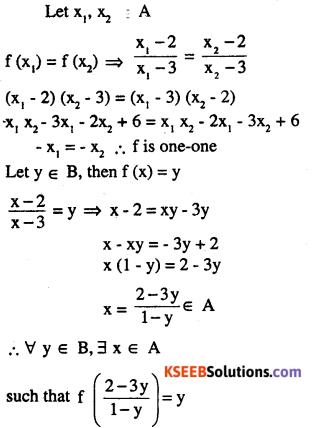Question 11.
Let f : R → R be defined as f(x) = x4. Choose the correct answer.
(A) f is one-one onto
(B) f is many-one onto
(c) f is one-one but not onto
(D) f is neither one-one nor onto.
f (x,) = f (x2) ⇒ x14 = x24
= x14 = x24 = 0
= (x1 – x2) (x1 + x2) (x12 = x22)
x1 = x2 or x1 = – x2
∴ f is not one-one
f (x) = x4 ≥ o ∀ x ∈ R
Range of f = [0, ∞) ∈ R
hence not onto